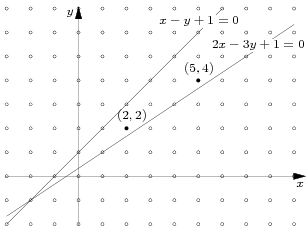시간 제한 메모리 제한 제출 정답 맞은 사람 정답 비율
1 초 128 MB 0 0 0 0.000%

## 문제

We are given six integers: A1, B1, C1, A2, B2, C2 such that A1B2A2B1. These numbers are coefficients in equations of two intersecting lines:

• l1: A1x + B1y + C1 = 0,
• l2: A2x + B2y + C2 = 0.

The lines divide the plane into four parts. We represent each part by any point with integer coordinates, belonging to this part (but not belonging to any of the lines l1, l2). You are given a point (a, b) with integer coordinates representing one part. Find a point with integer coordinates (c, d) representing the same part, such that its distance from the point of intersection of lines l1 and l2 is least possible.

Write a program which:

• reads the equations of lines l1 and l2 and a point representing one part of plane,
• finds a point with integer coordinates representing the given part and closest to the point of intersection of lines l1 and l2,
• writes the answer to the standard output.

## 입력

The first line of the standard input contains three numbers A1, B1, C1, separated by single spaces - the coefficients of l1's equation. The second line contains three numbers A2, B2, C2, separated by single spaces - the coefficients of l2's equation. It is true that A1B2A2B!. The third and last line contains two integers a, b, separated by a single space. They are the coordinates of a point representing one part of plane. Point (a, b) does not belong to any of lines l1, l2. For every number x from the input it is true that -2 100 000 000 < x < 2 100 000 000.

## 출력

Your program should write to the standard output two numbers c, d, separated by a single space - the coordinates of a point (c, d) representing the given part, closest to the point of intersection of lines l1 and l2. If there are many such points, your program should output only one of them.

## 예제 입력 1

1 -1 1
2 -3 1
5 4


## 예제 출력 1

2 2


## 힌트